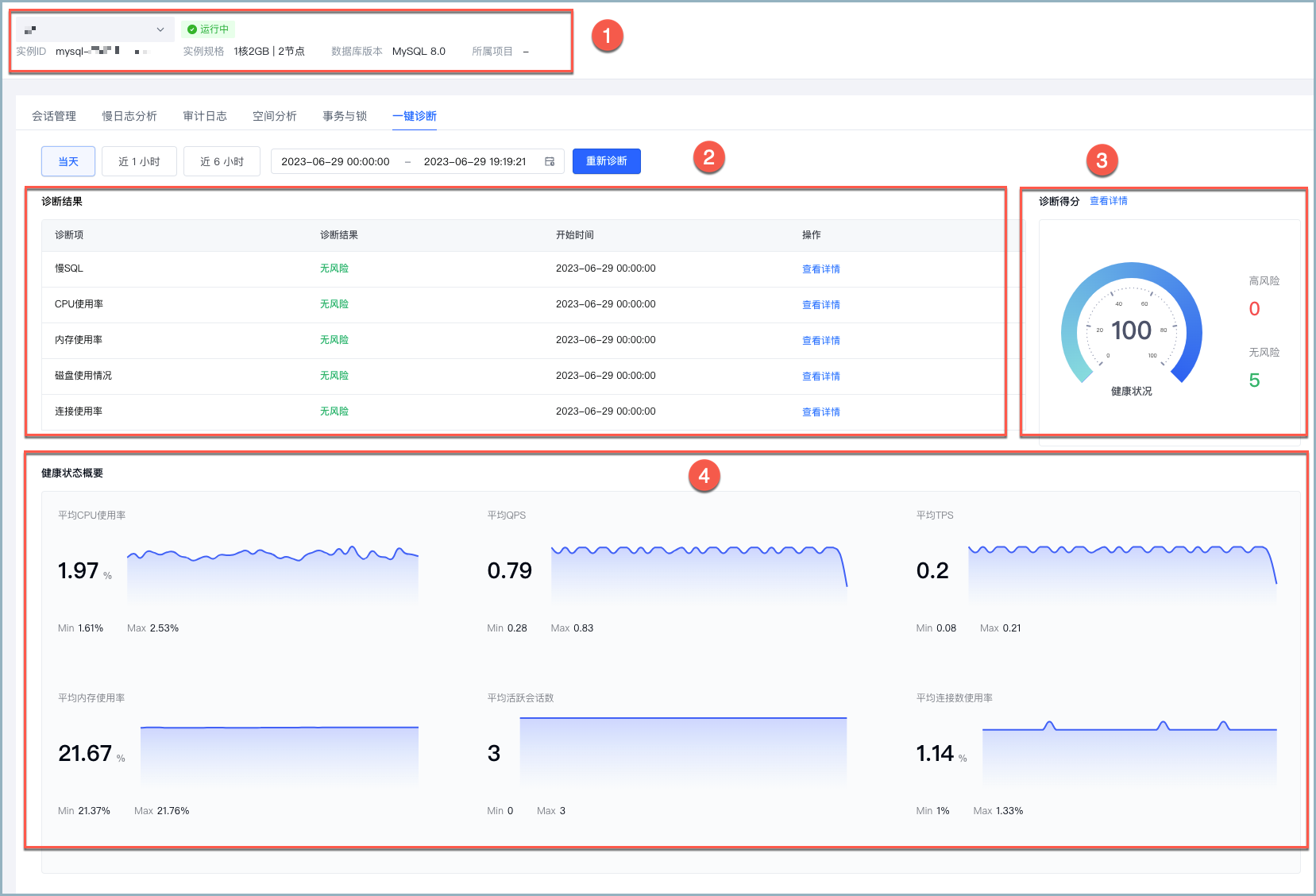You need to enable JavaScript to run this app.

# 一键诊断

## 注意事项

• 扣分规则

诊断项规则计算方式

CPU 使用率

按照 CPU 使用率进行扣分，最大可扣 10 分。

• CPU 使用率 < 70% 时，不扣分。

• CPU 使用率 >= 70% 时，扣分计算公式为：`min((使用率-70)*40/100, 10)`

内存使用率

按照内存使用率进行扣分，最大可扣 10 分。

• 内存使用率 < 70% 时，不扣分。

• 内存使用率 >= 70% 时，扣分计算公式为：`min((使用率-70)*40/100, 10)`

连接数使用率

连接使用率进行扣分，最大可扣 10 分。

• 连接数使用率 < 70% 时，不扣分。

• 连接数使用率 >= 70% 时，扣分计算公式为：`min((使用率-70)*40/100, 10)`

慢日志

按照每 24 小时慢日志的数量进行扣分，最大可扣 10 分。

• 慢日志数量 = 0 时，不扣分。

• 0 条 < 慢日志数量 < 50 条时，扣 1 分。

• 慢日志数量 >= 50 条时，扣分计算公式为 `int(min(数量/50, 10))`

磁盘使用率

剩余磁盘预计可使用天数进行扣分，最大可扣 15 分。

• 通过可使用天数计算扣分

• 可使用天数 >= 15 天，不扣分。

• 可使用天数 < 15 天时，扣分计算公式为 `int(15 - 天数/3 )`

• 通过使用率计算扣分

• 磁盘使用率 < 70% 时，不扣分。

• 磁盘使用率 >= 70% 时，扣分计算公式为：`min((使用率-70)*40/100, 10)`

说明

在统计磁盘使用率的最终得分时，按照两种扣分方式中较高的进行扣分。

• 诊断结果风险等级规则

诊断项无风险低风险中风险高风险
CPU 使用率cpuUsage < 70%70% <= cpuUsage < 80%80% <= cpuUsage < 90%90% <= cpuUsage
内存使用率memUsage < 70%70% <= memUsage < 80%80% <= memUsage < 90%90% <= memUsage
连接数使用率connectionUsage < 70%70% <= connectionUsage < 80%80% <= connectionUsage < 90%90% <= connectionUsage
慢日志slowlogs = 00 <= slowlogs < 100100 <= slowlogs < 300300 <= slowlogs

磁盘使用率

• 15 <= days

• diskUsage < 70%

• 12 <= days < 15

• 70% <= diskUsage < 80%

• 7 <= days < 12

• 80% <= diskUsage < 90%

• 0 <= days < 7

• 90% <= diskUsage

## 一键诊断界面介绍